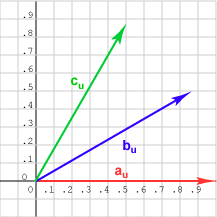Normalize the vectors (create unit vectors in the same directions).

# Normalized VectorsThe diagram shows each vector after it has been normalized. (Remember, this means creating a unit vector in the same direction as the original vector).

Recall that a subscript u indicates a unit vector: bu.

### QUESTION 4:

Now which dot product is larger?   au · bu ,  or au · cu  ?

Hint: remember that u · v   =   |u||v| cos θ. But now both vectors have length 1.0.